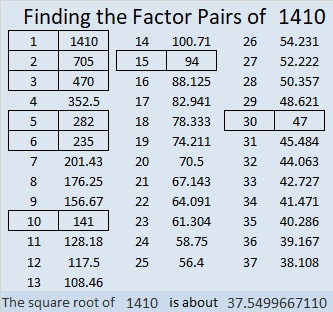# 1410 and Level 1

Start the school year off right with a quick review of the multiplication table. You can actually construct an entire 10 × 10 table with only the nine clues in this puzzle. Figure out where the numbers 1 to 10 go in both the first column and the top row and amaze yourself with how much you remember!Print the puzzles or type the solution in this excel file: 10 Factors 1410-1418

Here are some facts about the puzzle number, 1410:

• 1410 is a composite number.
• Prime factorization: 1410 = 2 × 3 × 5 × 47.
• 1410 has no exponents greater than 1 in its prime factorization, so √1410 cannot be simplified.
• The exponents in the prime factorization are 1, 1, 1, and 1. Adding one to each exponent and multiplying we get (1 + 1)(1 + 1)(1 + 1)(1 + 1) = 2 × 2 × 2 × 2 = 16. Therefore 1410 has exactly 16 factors.
• The factors of 1410 are outlined with their factor pair partners in the graphic below.1410 is the hypotenuse of a Pythagorean triple:
846-1128-1410 which is (3-4-5) times 282.